## Multiplication of money (1)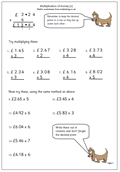Here we have a straightforward worksheet dealing with the multiplication of money by a single digit.The main concern here is that the process is carried out much as multiplying a 3 or 4-digit number, but remembering the decimal point.

The second set of questions, from 9. to 15 should be set out in the correct way and not attempted as shown.

It is often a good idea for children to check the answers to these types of page by using a calculator.

Multiplication of money (pg 1)

## Resource of the Week: short multiplication worksheets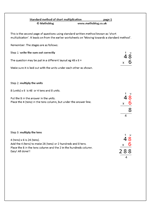‘Short’ multiplication, as it is known, means to use pencil and paper methods to multiply a 2-digit (or more) number by a one digit number in a vertical way.

In English schools children have plenty of experience building up to this method, in the hope that they understand what is going on. Unfortunately they are sometimes taught so many methods that they get totally confused.

These pages show simply, step by step how to carry out the method on paper, but a good knowledge of ‘times tables’ is needed.

Go to our resources on short multiplication.

## Multiplying decimals 1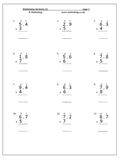The standard short method of multiplication can be used when multiplying a decimal by a single digit. Children need plenty of practice with this before moving on to working with decimals.

The key to success with these is to place the answer in the correct columns and the best bet is to put the decimal point in first. Think of the decimal point as a fixed point, therefore the point in the answer should be immediately above the point in the question. Apart from that, these should prove to be straightforward.

This maths worksheet shows the method and then gives 12 questions to practice.

Multiplying decimals (pg 1)

## Standard method of short multiplication pg 2Here is another maths worksheet to practice the standard method known as short multiplication. All these sums have already been set out in the correct way but it is important to remember to keep the units in the units, tens in the tens column etc. This will become even more important when decimals are introduced later.

Always work in the same order, starting by multiplying the units, carrying any tens on into the tens column and then multiplying the tens. Perhaps the key to success with these is knowing the times tables up to 10 times 10. If each multiplication has to be worked out the whole task becomes laborious.

Standard method of short multiplication pg 2

## Maths worksheet: Standard short multiplication 1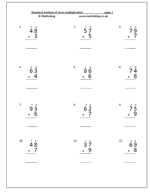This is the standard written method known as ‘short multiplication’. It leads on from the earlier worksheets on ‘Moving towards a standard method’.

Before starting this type of sum a good knowledge of times tables is needed.

The stages are as follows:

#### Step 1: write the sum out correctly

The question may be put in a different layout eg 38 x 7 =

Make sure it is laid out with the units under each other as shown on the example.

#### Step 2: multiply the units

Multiply 8 by 7 which 56 or 5 tens and 6 units.

Put the 6 in the answer in the units and place the 5 (tens) in the tens column, under the answer line. Continue reading “Maths worksheet: Standard short multiplication 1”

## Year 4 maths worksheet: multiplying by 10 and 100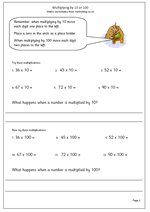Understanding what happens when you multiply a whole number by 10 is crucial to understanding maths at primary school and indeed with later maths. Children should have carried out lots of practical work with base 10 apparatus in school to show that:

when you multiply a whole number by 10 the digits move one place to the left.

Now this may give the appearance of ‘adding a nought’ as a zero is placed in the units. I know of many teachers and publishers who still use this expression, but it is extremely dangerous, especially when it comes to multiplying decimals by 10. (0.8 is not 0.80).

Multiplying by 100 is the same as multiplying by 10 and then multiplying by 10 again (10 x 10 = 100) so each digit will move two places to the left and zeros will need to be placed in the units and tens.

When you multiply a whole number by 100 the digits move two places to the left.

Multiplying by 10 and 100 (pg 1)

## Year 5 Maths Worksheet: Multiplying a 3-digit number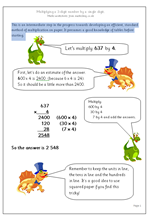This is an intermediate step in the progress towards developing an efficient, standard method of multiplication on paper. It presumes a good knowledge of times tables before starting.

An important part of this process which is often neglected, is that an estimate of the answer should be quickly worked out ‘in your head’ before beginning the written answer. This can be done by quickly rounding the numbers.

For example: 637 x4 is round about 2400.

This intermediate process does the sum 637 x 4 in four parts:

1. Multiply the hundreds 600 x 4

2.Multiply the tens 30 x 4

3. Multiply the units 7 x 4

The first three stages can be reversed, multiplying the units first.

Multiply a 3-digit number by a 1-digit number

## Free maths worksheet: Division and multiplication corresponding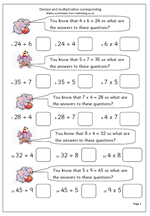This maths worksheet looks at the correspondence between multiplication and division. Given one fact, three others can be worked out. For example:

Given that  5 x 4 = 20 then:

a. 4 x 5 = 20

b. 20 ÷ 5 = 4

c. 20 ÷ 4 = 5

A full understanding of this relationship is needed before children can go on to written methods of division. If your child is struggling with division it would be well worth checking that they understand this.

To make sense of this it is a good idea to ‘talk through’ what these number sentences say.

If 5 lots of 4 are 20 then 4 lots of 5 will also be 20.

If 20 sweets are shared equally between 4 people they get 5 each.

If 20 sweets are shared equally between 5 people they get 4 each.

Multiplication and division corresponding

## Maths worksheet: Moving towards standard method for multiplication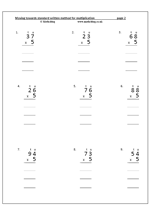This method of multiplication encourages understanding of the process of multiplying a 2-digit number by  a 1-digit number. The questions on this page would not normally be completed using written methods: mental methods should suffice. It would be expected that children could do these ‘in their heads’ from a  knowledge of multiplying by 10 and halving. Also, the Primary Framework for Mathematics does not recommend using these types of written method until Year 3 onwards (7/8 years old).

The method is to multiply the units first, then multiply the tens, finally adding the two together. It is a ‘half-way house’ towards the standard written method, explaining what happens when the tens boundary is crossed during multiplying the units (ie when the units come to more than 10). More details are shown on the worksheet pages below.

The stages are as follows:

36 x 5

Step 1: multiply the units

6 x 5 = 30

Put the 30 in the row below the question, making sure the 3 digit is in the tens column.

Step 2: multiply the tens

3 (tens) x 5  is 15 (tens) = 150.

Put the 150 in the row below, again making sure the units and tens
line up.